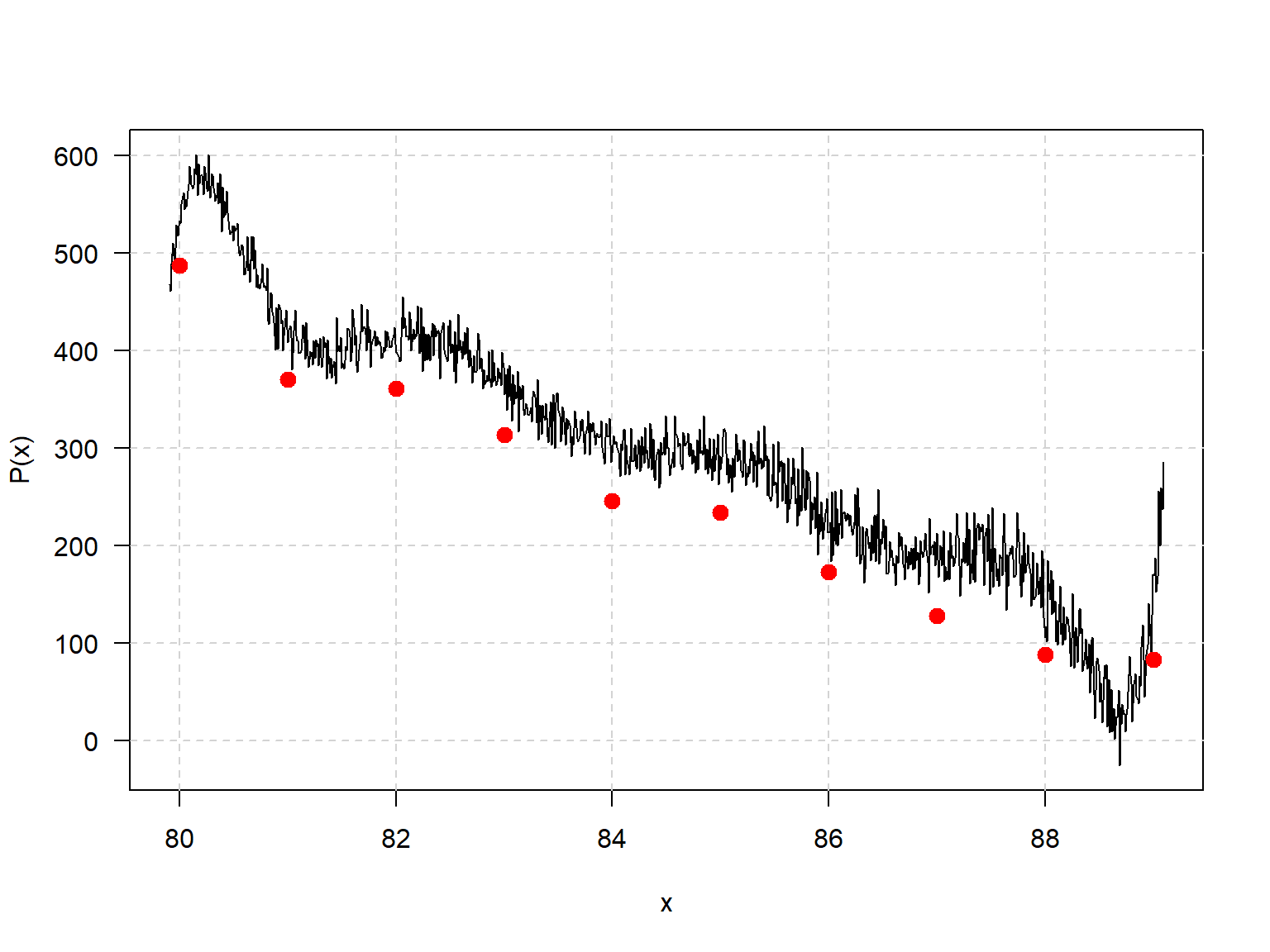## Preamble

The polynom package is an R collection of functions to implement a class for univariate polynomial manipulations. It is based on the corresponding S package by Bill Venables <Bill.Venables@gmail.com>, and was adapted to R by Kurt Hornik <Kurt.Hornik@R-project.org> and Martin Maechler <maechler@stat.math.ethz.ch>.

This document is based on the original ‘NOTES’, with minor updates.

# A Univariate Polynomial Class for R

## Introduction and summary

The following started as a straightforward programming exercise in operator overloading, but seems to be more generally useful. The goal is to write a polynomial class, that is a suite of facilities that allow operations on polynomials: addition, subtraction, multiplication, “division”, remaindering, printing, plotting, and so forth, to be conducted using the same operators and functions, and hence with the same ease, as ordinary arithmetic, plotting, printing, and so on.

The class is limited to univariate polynomials, and so they may therefore be uniquely defined by their numeric coefficient vector. Coercing a polynomial to numeric yields this coefficient vector as a numeric vector.

For reasons of simplicity it is limited to REAL polynomials; handling polynomials with complex coefficients would be a simple extension. Dealing with polynomials with polynomial coefficients, and hence multivariate polynomials, would be feasible, though a major undertaking and the result would be very slow and of rather limited usefulness and efficiency.

## General orientation

The function polynomial() creates an object of class polynomial from a numeric coefficient vector. Coefficient vectors are assumed to apply to the powers of the carrier variable in increasing order, that is, in the truncated power series form, and in the same form as required by polyroot(), the system function for computing zeros of polynomials. (As a matter or terminology, the zeros of the polynomial $$P(x)$$ are the same as the roots of equation $$P(x) = 0$$.)

Polynomials may also be created by specifying a set of (x, y) pairs and constructing the Lagrange interpolation polynomial that passes through them (poly.calc(x, y)). If y is a matrix, an interpolation polynomial is calculated for each column and the result is a list of polynomials (of class polylist).

The third way polynomials are commonly generated is via its zeros using poly.calc(z), which creates the monic polynomial of lowest degree with the values in z as its zeros.

The core facility provided is the group method function Ops.polynomial(), which allows arithmetic operations to be performed on polynomial arguments using ordinary arithmetic operators.

## Examples

1. Find the Hermite polynomials up to degree 5 and plot them. Also plot their derivatives and integrals on separate plots.

The polynomials in question satisfy \begin{aligned} He_0(x) &= 1,\\ He_1(x) &= x,\\ He_n(x) &= x He_{n-1}(x) - (n - 1) He_{n-2}(x), \qquad n = 2, 3, \ldots \end{aligned}

He <- list(polynomial(1), polynomial(0:1))
x <- polynomial()
for (n in 3:6) {
He[[n]] <- x * He[[n-1]] - (n-2) * He[[n-2]] ## R indices start from 1, not 0
}
He <- as.polylist(He)
plot(He)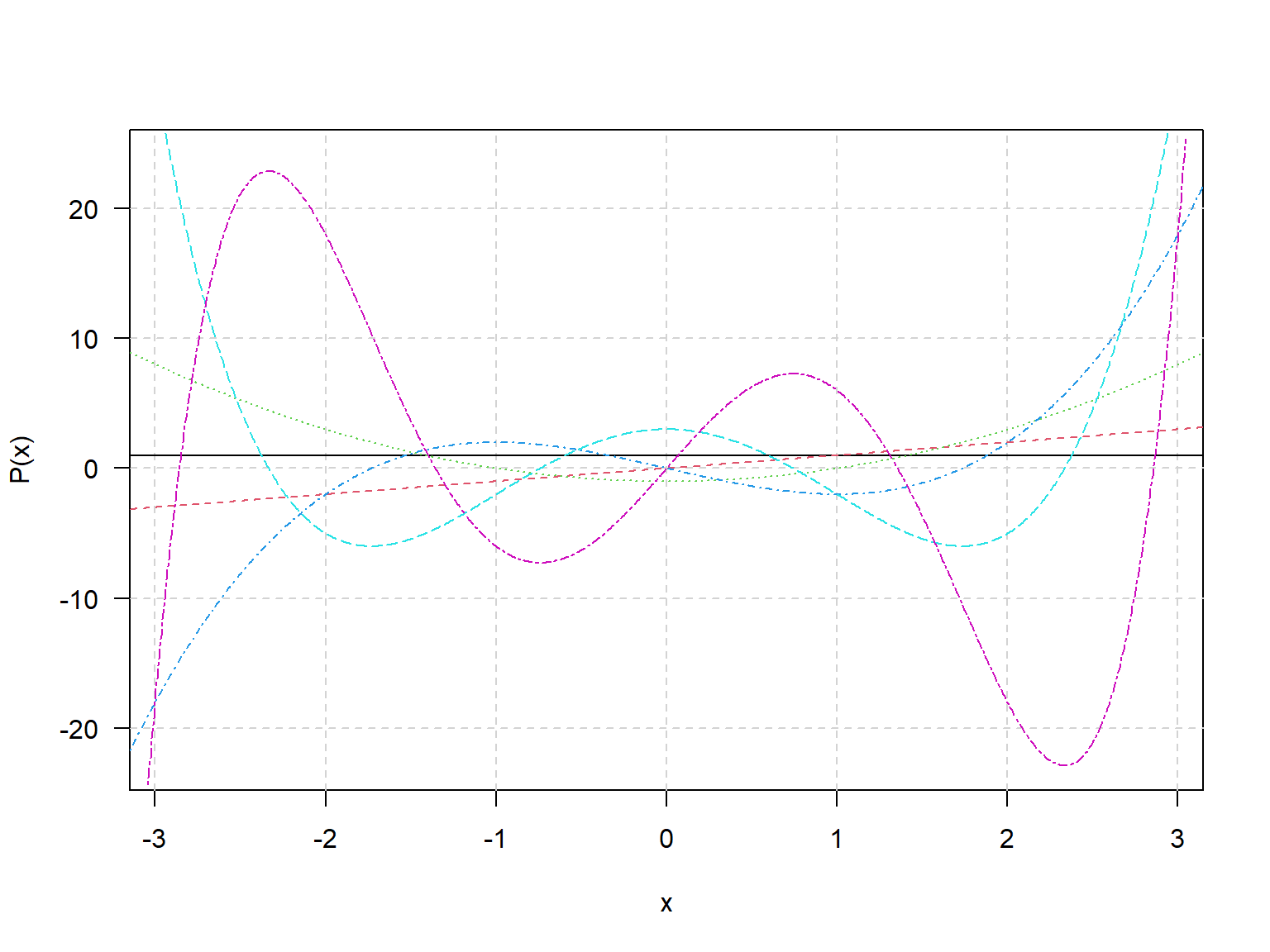plot(deriv(He))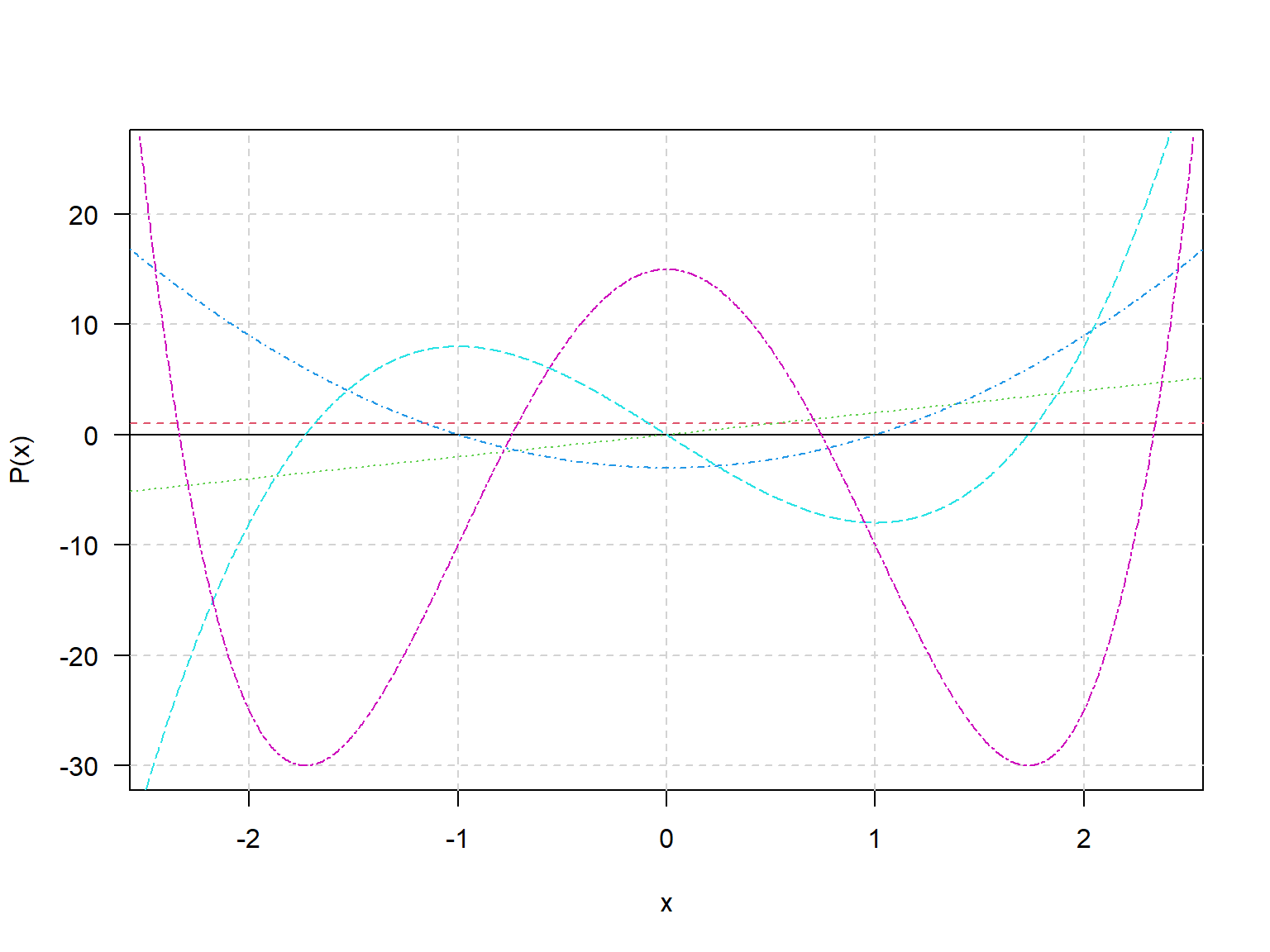plot(integral(He))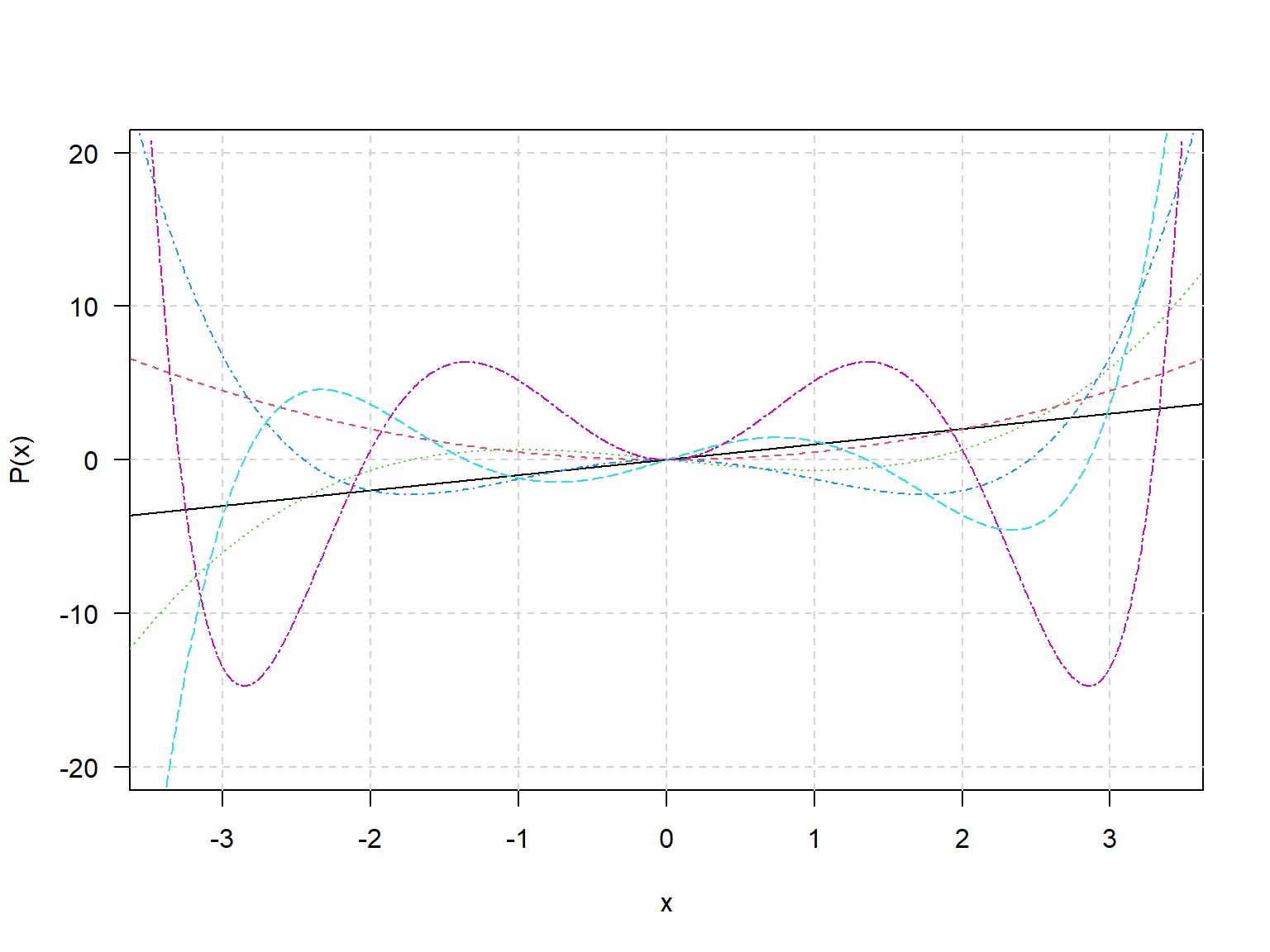1. Find the orthogonal polynomials on $$x = (0, 1, 2, 4)$$ and construct R functions to evaluate them at arbitrary $$x$$ values.
x <- c(0,1,2,4)
(op <- poly.orth(x))
List of polynomials:
[]
0.5

[]
-0.591608 + 0.3380617*x

[]
0.5640761 - 1.168443*x + 0.282038*x^2

[]
-0.2860388 + 3.114644*x - 2.502839*x^2 + 0.4370037*x^3
(fop <- lapply(op, as.function))
[]
function (x)
{
w <- 0
w <- 0.5 + x * w
w
}
<environment: 0x00000000159b7880>

[]
function (x)
{
w <- 0
w <- 0.338061701891407 + x * w
w <- -0.591607978309962 + x * w
w
}
<environment: 0x00000000159bdf80>

[]
function (x)
{
w <- 0
w <- 0.282038037408883 + x * w
w <- -1.1684432978368 + x * w
w <- 0.564076074817766 + x * w
w
}
<environment: 0x00000000159bec40>

[]
function (x)
{
w <- 0
w <- 0.437003686737563 + x * w
w <- -2.50283929676968 + x * w
w <- 3.11464445820226 + x * w
w <- -0.286038776773677 + x * w
w
}
<environment: 0x00000000159c5298>
(P <- sapply(fop, function(f) f(x)))
[,1]        [,2]       [,3]        [,4]
[1,]  0.5 -0.59160798  0.5640761 -0.28603878
[2,]  0.5 -0.25354628 -0.3223292  0.76277007
[3,]  0.5  0.08451543 -0.6446584 -0.57207755
[4,]  0.5  0.76063883  0.4029115  0.09534626
zapsmall(crossprod(P))     ### Verify orthonormality
[,1] [,2] [,3] [,4]
[1,]    1    0    0    0
[2,]    0    1    0    0
[3,]    0    0    1    0
[4,]    0    0    0    1
1. Miscellaneous computations using polynomial arithmetic.
(p1 <- poly.calc(1:6))
720 - 1764*x + 1624*x^2 - 735*x^3 + 175*x^4 - 21*x^5 + x^6
(p2 <- change.origin(p1, 3))
-12*x + 4*x^2 + 15*x^3 - 5*x^4 - 3*x^5 + x^6
predict(p1, 0:7)
 720   0   0   0   0   0   0 720
predict(p2, 0:7)
     0     0     0     0   720  5040 20160 60480
predict(p2, 0:7 - 3)
 720   0   0   0   0   0   0 720
(p3 <- (p1 - 2 * p2)^2)         # moderate arithmetic expression.
518400 - 2505600*x + 5354640*x^2 - 6725280*x^3 + 5540056*x^4 - 3137880*x^5 +
1233905*x^6 - 328050*x^7 + 53943*x^8 - 4020*x^9 - 145*x^10 + 30*x^11 + x^12
fp3 <- as.function(p3)          # should have 1, 2, 3 as zeros
fp3(0:4)
  518400       0       0       0 2073600
1. Polynomials can be numerically fragile. This can easily lead to surprising numerical problems.
x <- 80:89
y <- c(487, 370, 361, 313, 246, 234, 173, 128, 88, 83)

p <- poly.calc(x, y)        ## leads to catastropic numerical failure!
predict(p, x) - y
 38.5 45.5 44.0 16.0 90.0 96.5 62.0 34.5 -2.5 28.0

p1 <- poly.calc(x - 84, y)  ## changing origin fixes the problem
predict(p1, x - 84) - y
  7.389644e-12  1.989520e-12  3.410605e-13  0.000000e+00  0.000000e+00
  5.684342e-14  1.421085e-13 -2.842171e-14 -3.296918e-12 -2.199840e-11

plot(p1, xlim = c(80, 89) - 84, xlab = "x - 84")
points(x - 84, y, col = "red", cex = 2)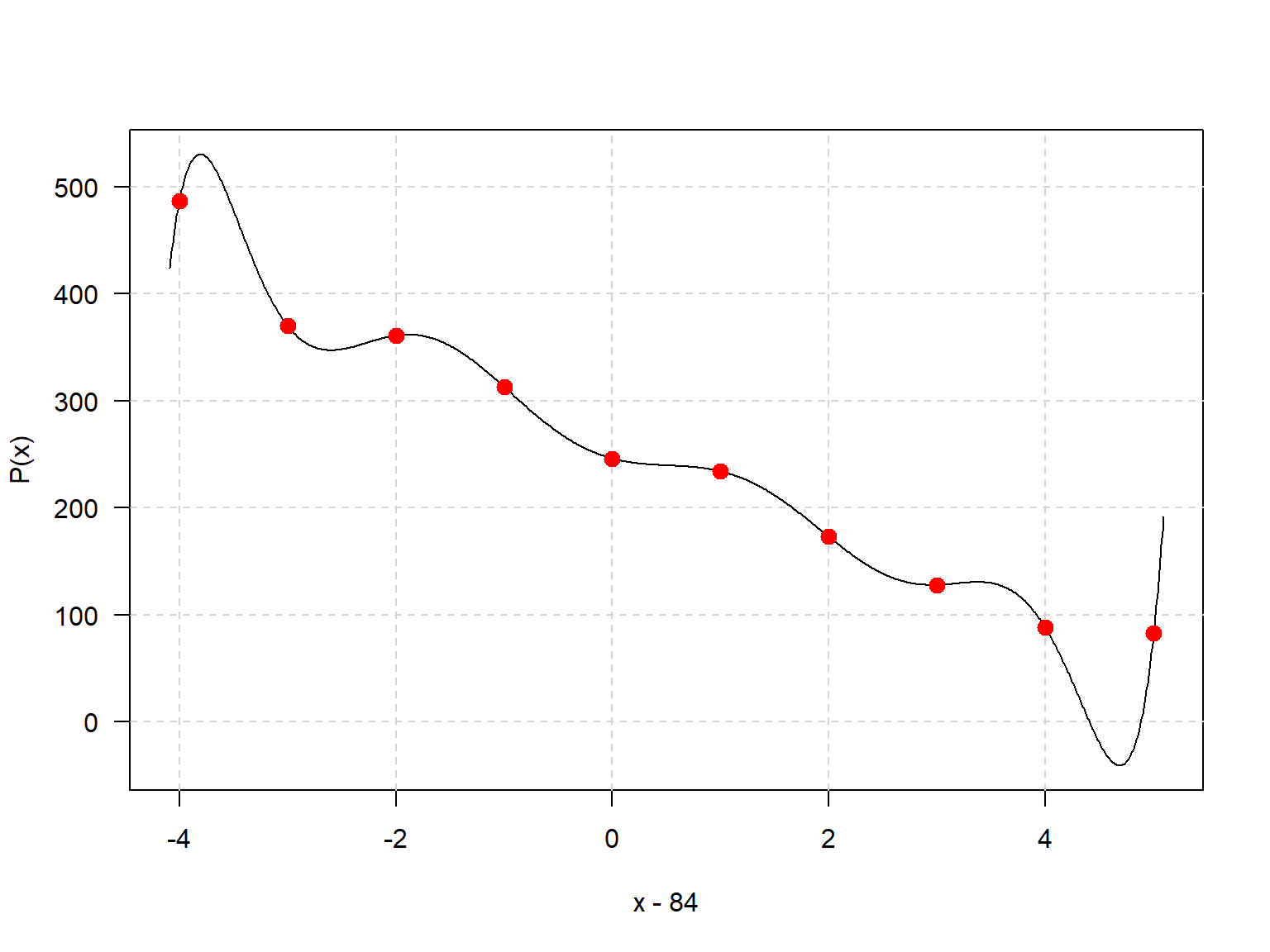#### Can we now write the polynomial in "raw" form?

p0 <- as.function(p1)(polynomial() - 84) ## attempt to change the origin back to zero
points(x, y, col = "red", cex = 2)  ## major numerical errors due to finite precision